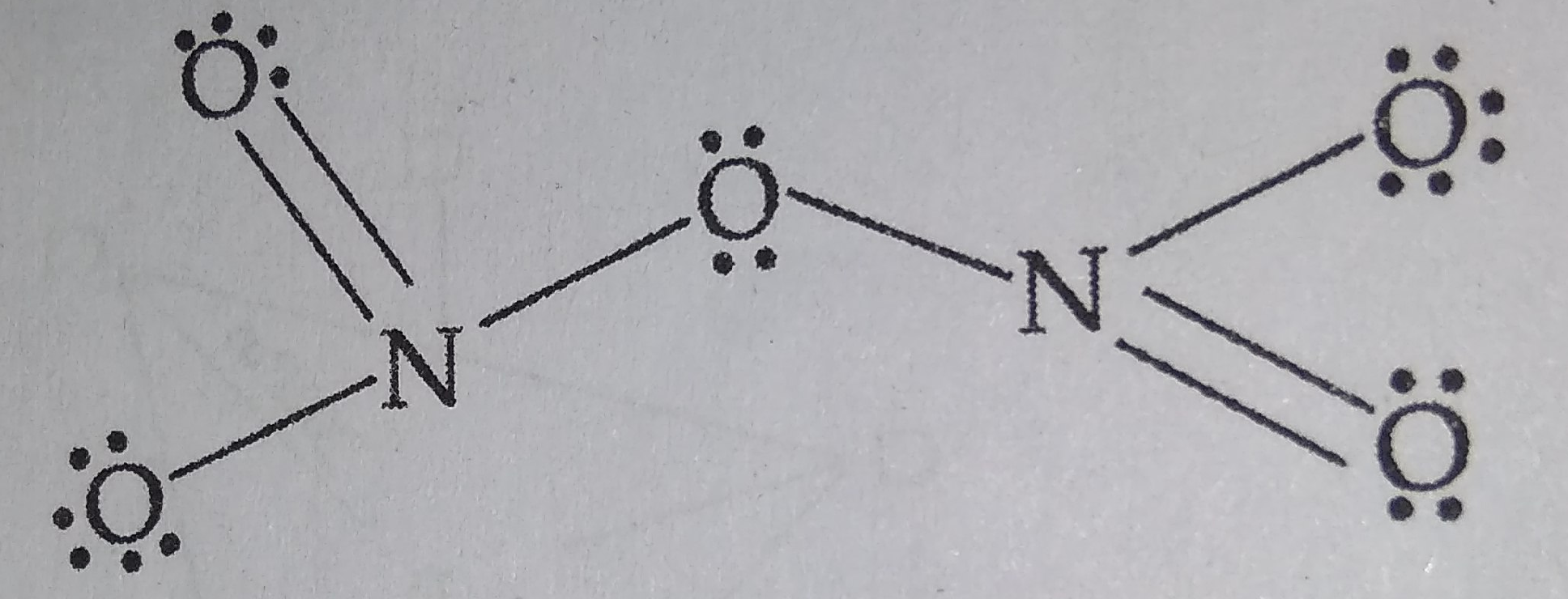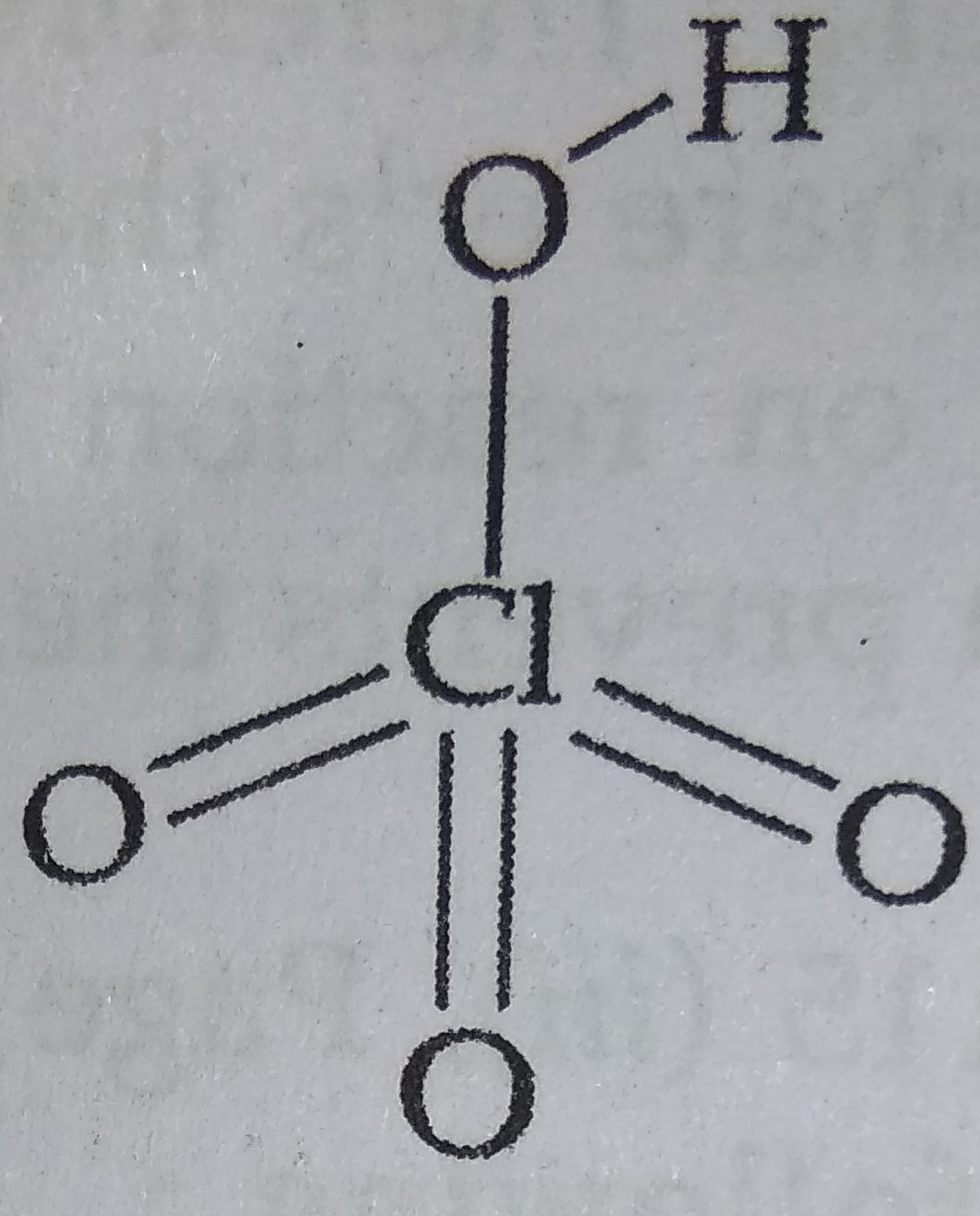# NEET Chemistry The p-Block Elements (XII) Questions SolvedBOARD

(a) Draw the structures of the following molecules :

(i) ${N}_{2}{O}_{5}$                             (ii) $HCI{O}_{4}$

(b) Explain the following observations :

(i) ${H}_{2}S$ is more acidic than ${H}_{2}O.$

(ii) Fluorine does not exhibit any positive oxidation state.

(iii) Helium forms no real chemical compound.

Concept Videos :-

#2 | 15th Group: Properties: II
#3 | 15th Group: Ammonia

Concept Questions :-

group 15 ,preparation and properties

(a) (i) ${N}_{2}{O}_{5}$ :Planar

(ii) $HCI{O}_{4}$ :(b) (i) ${H}_{2}S$ is more acidic than ${H}_{2}O$ because bond dissociation enthalpy of H-S bond in ${H}_{2}S$ is less than that of H-O bond in ${H}_{2}O.$

(ii) F is the most electronegative element. It has no d-orbitals and therefore, there is no scope for any electron promotion. So it can only show oxidation state of -1 in its compounds.

(iii) Helium has very high ionisation enthalpy and no vacant d-orbitals, therefore no chemical compound of helium is known.

Difficulty Level:

Crack NEET with Online Course - Free Trial (Offer Valid Till September 22, 2019)